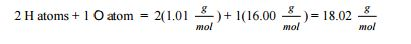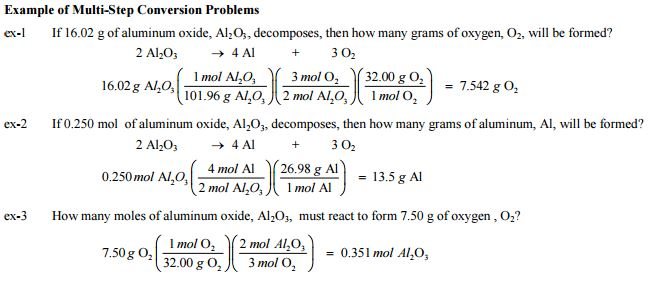# Mole concept and molar mass

The nature of chemistry is to change one molecule into one or more new molecules in order to create new substances such as paints, fertilizers, food additives, medicines, etc. When a chemist is performing a reaction to make the new product, the chemist must know how much of the starting reactants to place in the reaction vessel. You know from your experience in the lab, that you can determine how much of a substance you have by determining the mass or volume of the substance.

However, atoms and molecules react not by mass or volume, but instead by number. This is exemplified when we write coefficients to balance chemical equations or subscripts in a chemical formula. These numbers are “counts” and have the unit of mole. A mole is a unit of counting similar to a dozen, except where a dozen is 12 of anything a mole is 6.022 x 1023 of anything.

This number is known as Avogadro’s number and is named after Amadeo Avogadro whose work in atomic molecular theory of gases led to the discovery of the number. Avogadro's number allows the chemist to determine the number of atoms or molecules in a sample. However, the measured size of a sample is still typically determined by mass on a balance. It is up to the chemist to interconvert between mass (g) and moles (the count).

This conversion is done with a conversion factor known as molar mass, M, which is the mass of 1mol and has the units of g/mol. Molar mass is determined by summing the atomic masses for each atom,### Examples of Mole